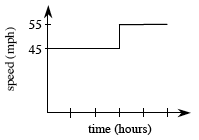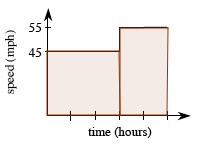### Home > PC > Chapter 4 > Lesson 4.1.1 > Problem4-12

4-12.

A car travels $45$ mph for $3$ hours and $55$ mph for the next two hours.

1. How far did the car travel?

2. Draw a speed vs. time graph of the situation.

3. What is the area under your curve from $0 ≤ h ≤ 5$?

4. How do your answers to part (a) and part (c) compare? Why?

distance = (rate)(time)

$(45)(3) + (55)(2)$

Added to graph, vertical segments from x axis to horizontal segments at x = 3, and x = 5, areas below horizontal segments are shaded.

They are the same because the area under the curve is the distance traveled.